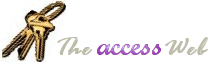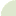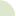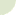Home  |   French  |   About  |   Search  | mvps.orgWhat's New Table Of Contents Credits Netiquette 10 Commandments  Bugs Tables Queries Forms Reports Modules APIs Strings Date/Time General Downloads Resources Search Feedback mvps.orgGeneral: Bits and Bitmasks Author(s) Terry KreftBitwise operations allow us to use one numeric field to represent several possible values in VBA.  This concept is similar to those familiar with the Win32 API; a lot of  API functions accept a flags argument which can represent a multitude of values internally to the function. 4 bits can store up to 16 numbers (for any one who feels like being picky I'm ignoring the possibility of using a sign bit). The reason why it can hold up to 16 numbers is because each of the 4 bits can be a 0 or a 1, as in ```0000, 0001, 0010, 0011, 0100, 0101, 0110, 0111, 1000, 1001, 1010, 1011, 1100, 1101, 1110, 1111``` If you think of this as a series of binary numbers you can see that they are the equivalents of the following decimal numbers:- ```0, 1, 2, 3, 4, 5, 6, 7, 8, 9, 10, 11, 12, 13, 14, 15``` If you consider the numbers where there is only a single 1 in the group of four characters, which are ```0001 (= 1) 0010 (= 2) 0100 (= 4) 1000 (= 8)``` Then by adding these numbers together we get one of the other possible numbers, e.g. ``` 1 + 2 = 3 (0001 + 0010 = 0011) 1 + 4 = 5 (0001 + 0100 = 0101) 4 + 8 = 12 (0100 + 1000 = 1100) and so on... 1 + 2 + 4 + 8 = 15 ( 0001 + 0010 + 0100 + 1000 = 1111)``` Now bitmasking involves using the AND operator to see which bits are set, so for example say you had the number 7 and you wanted to see if bit 1 was set (bit numbering starts at 0 so this is the second digit from the right in the binary numbers), you would do this `?(7 AND 2) = 2` This would return True, because bit 1 in the number 7 (0111) is set (i.e. it's a 1) The way it works is each bit in the first number is compared to the corresponding bit in the second number. If the bits are both 1 (i.e. they are both set) then it sets the corresponding bit in the result. If either (or both) of the bits is not set (i.e. it is a 0) then the corresponding bit in the result is not set (i.e. is a 0) If we look at the example above using bits then we see ``` 7 = 0111 2 = 0010 0th bit comparison 1 And 0 = 0 1st bit comparison 1 And 1 = 1 2nd bit comparison 1 And 0 = 0 3rd bit comparison 1 And 0 = 0``` Which when we lay it out horizontally is `0010 = 2` Therefore the bitwise comparison of 7 And 2 is 2 so `?(7 AND 2) = 2` results in True being returned. Lets take an example of using bitmasking to store results, let's say we're running an employment agency and we want to store four properties about applicants, these being their sex, are they employed, can they drive and are they looking for full time employment. We'll assign some numbers to these "properties" of our clients  ``` Const IsFemale = 1 Const IsEmployed = 2 Const CanDrive = 4 Const FullTime = 8``` When we get a new applicant we ask them the relevant questions and assign the corresponding numbers. Let's say we get a new client Fred, he is Male, Employed, he can't drive and he's looking for full time work, that is `IsEmployed + FullTime (= 2 + 8 = 10)` We therefore store the number 10 against this client in the table (lets say in the AppProps field), later on we could do a bitwise comparison of our clients. To find out who is employed, for Fred's record we would get `?(AppProps and FullTime) = FullTime` Would return True, showing that he is employed, but let's say the work involved required a woman, then `?(AppProps AND IsFemale) = IsFemale` Would return False, so we would know that Fred was not suitable for the job.  Alternatively we could do this in one operation, i.e. `?(AppProps And (IsFemale Or FullTime)) = (IsFemale Or FullTime) ` Which would return False as well. Let's take another Client Joan, Employed, is FullTime and Female, so her AppProps field contains the number 11 `(IsEmployed + FullTime + IsFemale = 2 + 8 + 1 = 11)` For Joan we get, `?(AppProps and FullTime) = FullTime` returns True `?(AppProps AND IsFemale) = IsFemale` returns True, and `?(AppProps And (IsFemale Or FullTime)) = (IsFemale Or FullTime)` returns True. So Joan is suitable for the job. Here's a bit of code to illustrate this: ```'************* Code Start ******************** Const IsFemale = 1 Const IsEmployed = 2 Const CanDrive = 4 Const FullTime = 8 Function TestBitMasks() Dim FredProps As Byte Dim JoanProps As Byte FredProps = IsEmployed + FullTime Debug.Print "Fred is FullTime?: "; _ (FredProps And FullTime) = FullTime Debug.Print "Fred is Female?: "; _ (FredProps And IsFemale) = IsFemale Debug.Print "Fred is FullTime and Female?: "; _ (FredProps And (IsFemale Or FullTime)) = (IsFemale Or FullTime) JoanProps = IsEmployed + FullTime + IsFemale Debug.Print "Joan is FullTime?: "; _ (JoanProps And FullTime) = FullTime Debug.Print "Joan is Female?: "; _ (JoanProps And IsFemale) = IsFemale Debug.Print "Joan is FullTime and Female?: "; _ (JoanProps And (IsFemale Or FullTime)) = (IsFemale Or FullTime) End Function '************* Code End ********************```© 1998-2010, Dev Ashish & Arvin Meyer, All rights reserved. Optimized for Microsoft Internet Explorer